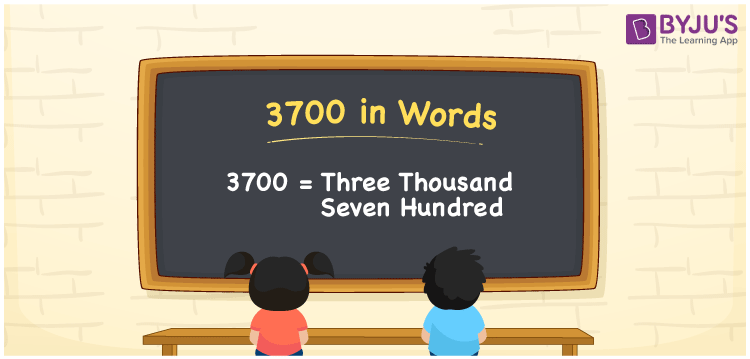# 3700 in Words

3700 in words is written as Three Thousand Seven Hundred. In Mathematics, numbers in words are written using the English alphabet. If you bought a cycle worth Rs. 3700, then you can write “I have bought a cycle worth Rupees Three Thousand Seven Hundred”. 3700 is a cardinal number as it denotes a specific amount. In this article, With the help of a place value chart, let us learn how to write 3700 in words.

 3700 in words Three Thousand Seven Hundred Three Thousand Seven Hundred in numerical form 3700

## 3700 in English Words

In Mathematics, we know that the English alphabet is used to write numbers in words. Hence, the English language is necessary for students to learn how to spell and write numbers in words. Thus, 3700 in words is written as Three Thousand Seven Hundred.## How to Write 3700 in Words?

3700 in words can be written by knowing the place value of its digits. Therefore, 3700 can be represented in the place value chart as:

 Thousands Hundreds Tens Ones 3 7 0 0

Therefore, we can write the expanded form as:

3 x Thousand + 7 x Hundred + 0 × Ten + 0 × One

= 3 x 1000 + 7 x 100 + 0 x 10 + 0 x 1

= 3000 + 700

= 3700

= Three Thousand Seven Hundred

Hence, 3700 in words is written as Three Thousand Seven Hundred.

Interesting way of writing 3700 in words

3 = Three

37 = Thirty-Seven

370 = Three Hundred and Seventy

3700 = Three Thousand Seven Hundred

Hence, the word form of the number 3700 is Three Thousand Seven Hundred.

3700 is a natural number that is succeeded by 3699 and preceded by 3701

• 3700 in words – Three Thousand Seven Hundred
• Is 3700 an odd number? – No
• Is 3700 an even number? – Yes
• Is 3700 a perfect square number? – No
• Is 3700 a perfect cube number? – No
• Is 3700 a prime number? – No
• Is 3700 a composite number? – Yes

## Frequently Asked Questions on 3700 in Words

Q1

### How to write 3700 in words?

3700 in words can be written as Three Thousand Seven Hundred.
Q2

### What is the value of 3700 minus 1950?

3700 minus 1950 = 3700 – 1950 = 1750 Hence, the value of 3700 minus 1950 is 1750.
Q3

### Is 3700 a composite number?

Yes, 3700 is a composite number since it has more than two factors.